# Open-loop Vector Control Technology for Synchronous Reluctance Motor

Because of its unique advantages compared with asynchronous motors and permanent magnet synchronous motors, it has a good application prospect in the field of traditional transmission and emerging electric vehicle driving.

Because of its unique advantages compared with asynchronous motors and permanent magnet synchronous motors, it has a good application prospect in the field of traditional transmission and emerging electric vehicle driving.

In terms of structure and principle, SynRM is more like a permanent magnet synchronous motor with permanent magnets removed. They have similar mathematical models. Therefore, for the SynRM drive mode: in hardware, fully compatible with the mature permanent magnet synchronous motor drive platform, reducing the cost of the synchronous reluctance motor drive control system. In software, according to the mathematical model characteristics of synchronous reluctance motor, a new flux observer is developed to realize the vector decoupling control of this kind of motor. Its open loop vector control block diagram is as follows.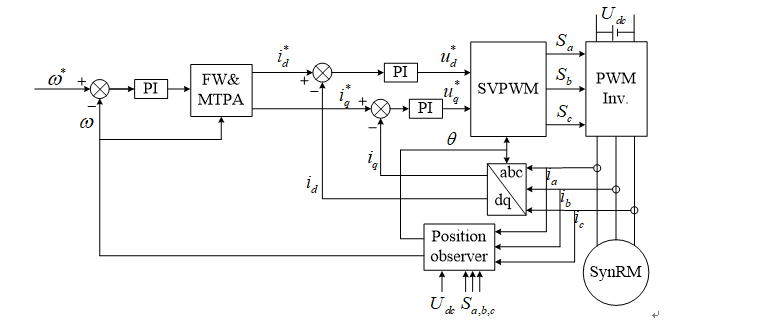Open loop vector control block diagram

Open-loop vector control can achieve better dynamic and static performance of synchronous reluctance motors, but has a strong dependence on motor parameters. The accuracy of the motor parameters in the model directly affects the reasonable design of the controller bandwidth, as well as the accuracy of flux linkage observation and velocity observation, which in turn affects the speed regulation performance of the system. The parameters of the synchronous reluctance motor are more severe than those of the permanent magnet synchronous motor, which poses a problem for control.

In view of the above problems and characteristics, our company has carried out in-depth research and optimization on the open-loop vector control technology of synchronous reluctance motor on AC310 platform, so as to obtain excellent dynamic and static performance. The specific significant results are as follows:

(1) Based on the existing leading vector control software platform, the new flux observer is used to achieve lower motor parameter sensitivity, accurate rotor position observation and accurate current decoupling.

(2) New motor inductance saturation characteristics learning. Since SynRM relies on reluctance torque operation, the motor magnetic circuit saturation phenomenon is obvious, and the motor inductance parameters are greatly affected by the magnetic circuit saturation. The direct-axis inductance Ld and the cross-axis inductance Lq are not fixed values, which seriously affect the transient response and precise control, so that the control performance of the motor cannot be optimized. In addition to the conventional self-learning, the AC310 has newly developed the inductance parameter saturation learning function, which has a short learning time and high learning precision. By learning, the d and q-axis inductance saturation characteristics of the motor can be obtained under different magnetic saturation levels, so that the motor can be more precisely controlled. The figure below shows the learning curve of the inductance saturation curve of a synchronous reluctance motor used in actual field.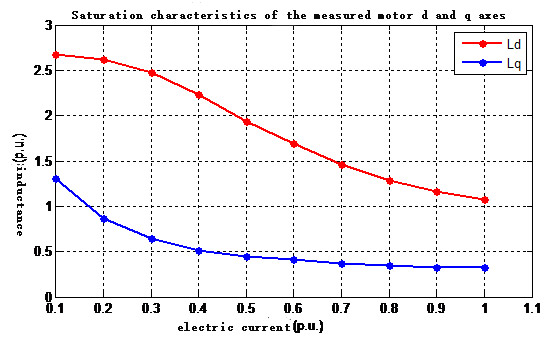New motor inductance saturation characteristics learning

(3) MTPA control. For a synchronous reluctance machine, there is no copper loss in the rotor without winding, and the loss is mainly concentrated on the stator side. The maximum torque-to-current ratio control is used to optimally distribute the dq-axis stator current to minimize the stator current, thereby minimizing the copper loss of the stator to achieve optimum efficiency.

According to the torque expression: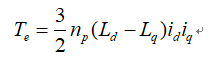If the inductance is considered to be constant, when θ = π / 4, the torque output at the same stator current is the largest. However, in the actual operation of the motor, the magnetic saturation has a great influence on the motor parameters. At this time, the optimal torque angle θ will deviate from π/4, and the optimal control of the efficiency cannot be achieved by using the constant current angle of 45°. Through the motor inductance saturation characteristics, the MTPA curve is set and the current angle is automatically adjusted, so that the optimal efficiency control is obtained at any frequency and load, and the energy efficiency of the synchronous reluctance motor is exerted to IE4 and above.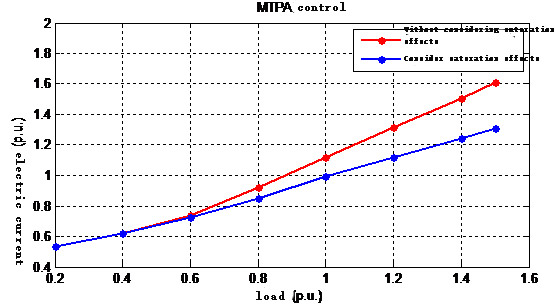MTPA control

(4) Field weakening control. The motor is subjected to MTPA control below the base speed, and the field weakening control is performed above the base speed to maximize the use of the voltage limit to maximize the output torque and expand the constant power range.

The following is a partial test waveform: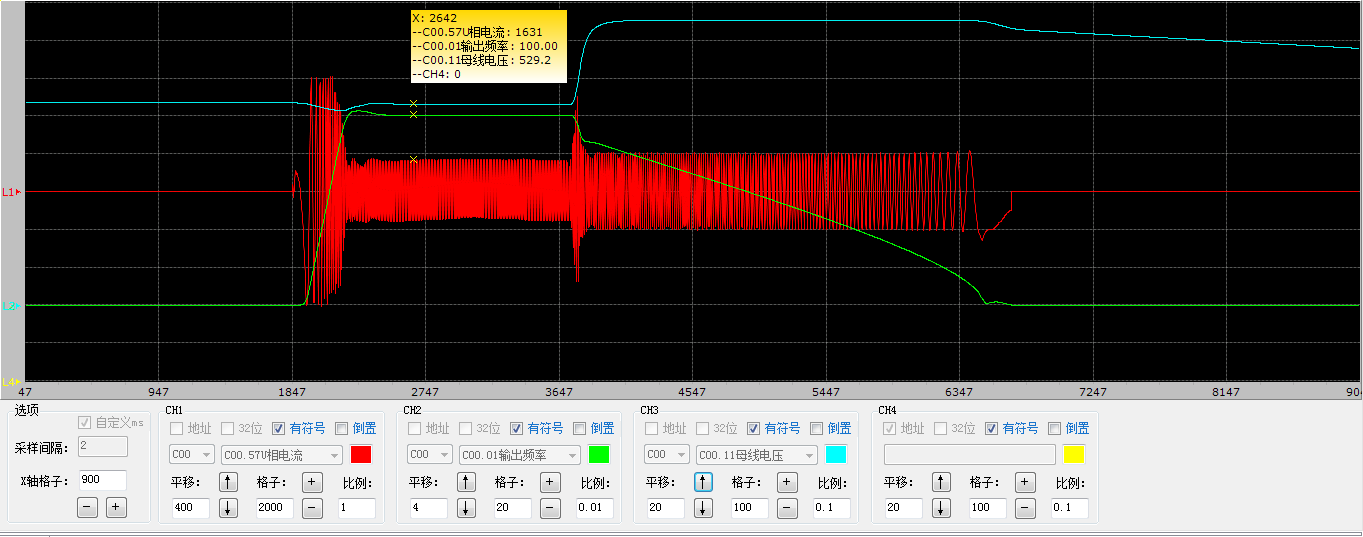Acceleration and deceleration time 0.1s, no-load acceleration from 0 to rated frequency and rapid deceleration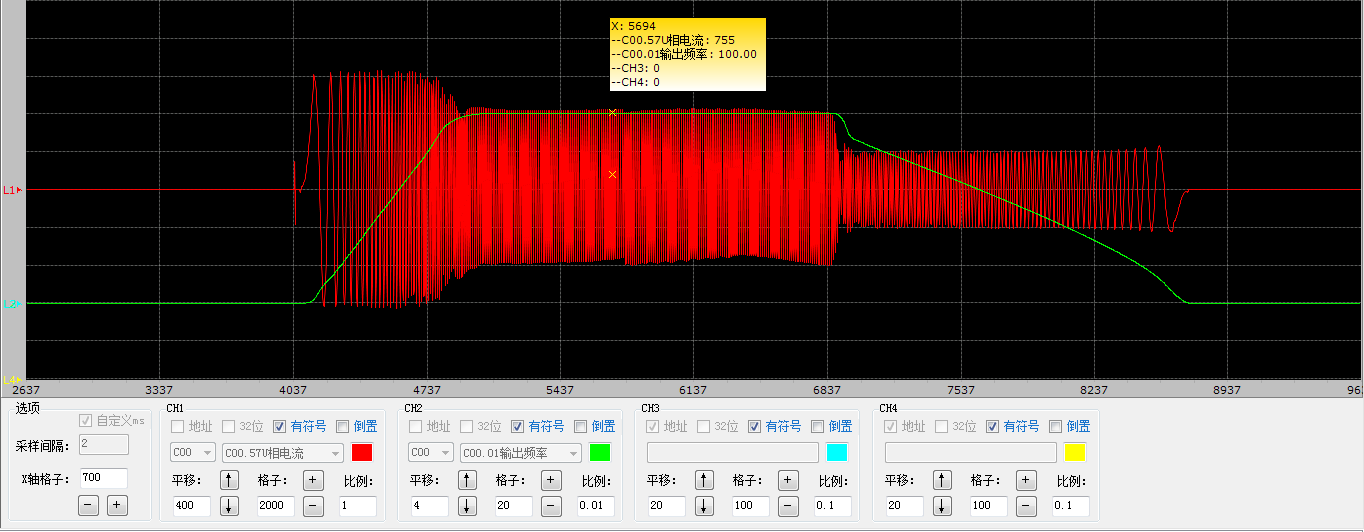Acceleration and deceleration time 0.1s, full load from 0 to rated frequency, rapid acceleration and rapid deceleration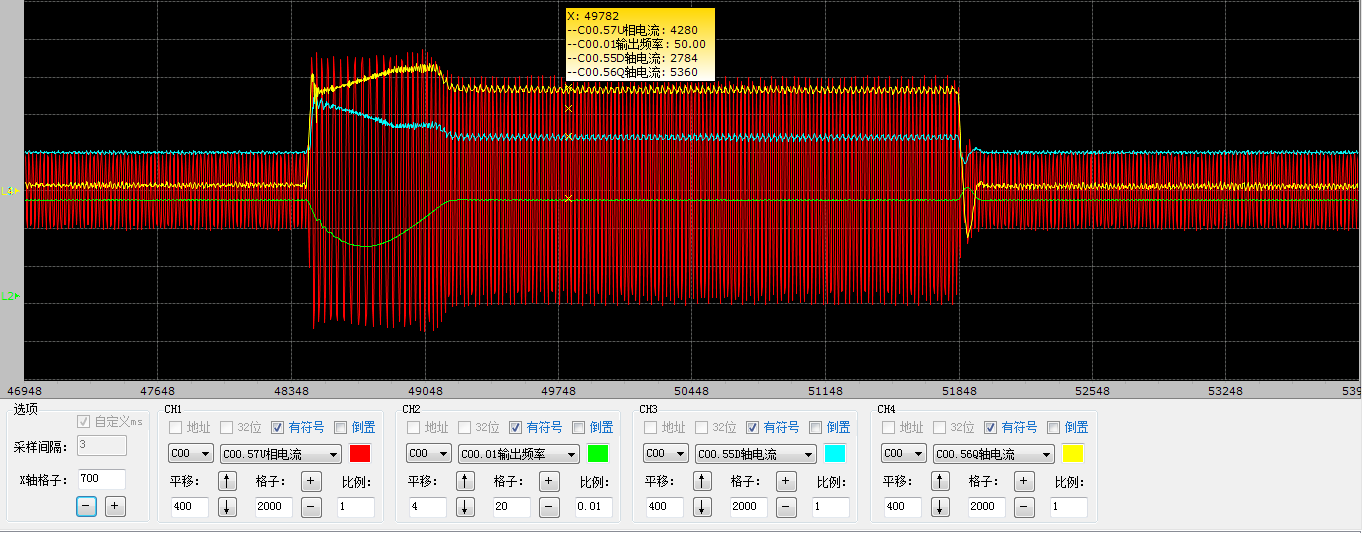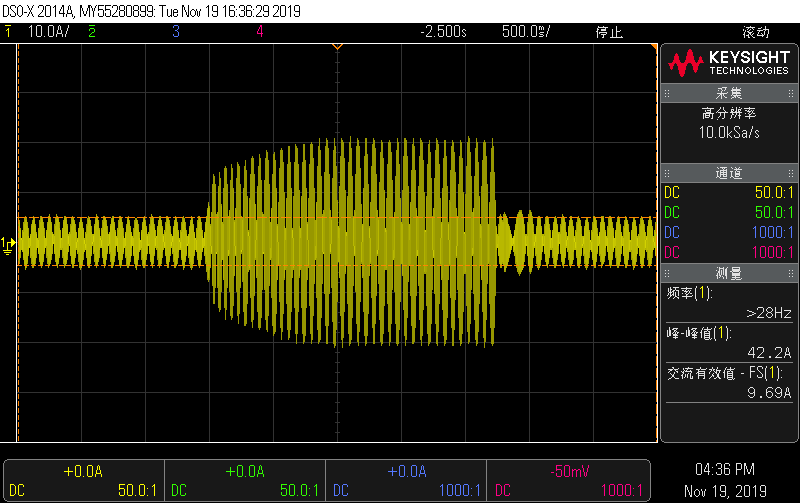1.3 times rated speed sudden increase, sudden discharge full load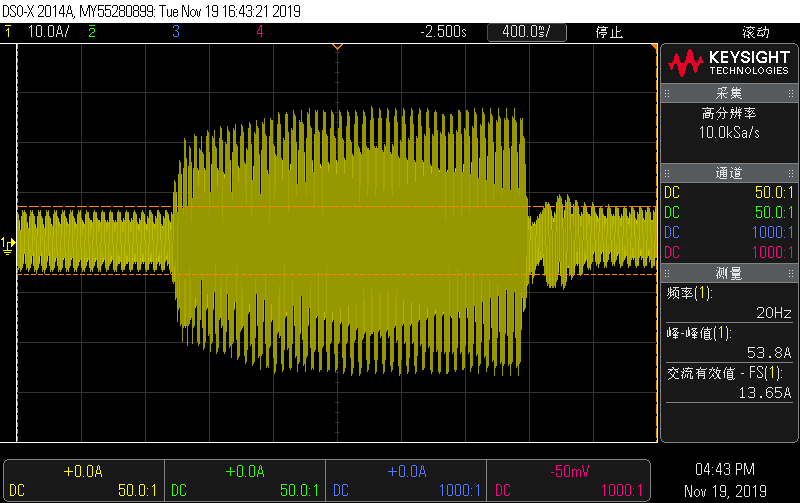Input voltage reduced by 20%, rated speed rated load limit test

### Summary

AC310 inverter synchronous reluctance motor open-loop vector control technology can drive synchronous reluctance motor stably and reliably, and has achieved excellent control effect. This is the first domestic generalized productization of synchronous reluctance motor control technology. Originated from the endless pursuit of driving, VEICHI will continue to provide customers with the latest and best electric drive solutions.